# Obtuse Triangle - Definition with Examples

The Complete K-5 Math Learning Program Built for Your Child

• 30 Million Kids

Loved by kids and parent worldwide

• 50,000 Schools

Trusted by teachers across schools

• Comprehensive Curriculum

Aligned to Common Core

## What is Obtuse Triangle?

An obtuse-angled triangle is a triangle in which one of the interior angles measures more than 90° degrees. In an obtuse triangle, if one angle measures more than 90°, then the sum of the remaining two angles is less than 90°.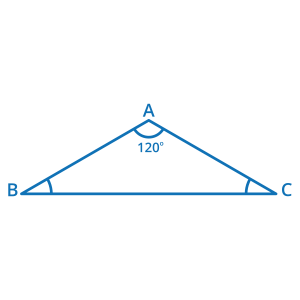Here, the triangle ABC is an obtuse triangle, as ∠A measures more than 90 degrees. Since, ∠A is 120 degrees, the sum of ∠B and ∠C will be less than 90° degrees.

In the above triangle, ∠A + ∠B + ∠C = 180° (because of the Angle Sum Property)

Since ∠A = 120°, therefore, ∠B + ∠C= 60°.

Hence, if one angle of the triangle is obtuse, then the other two angles with always be acute.

### Some examples of obtuse triangles: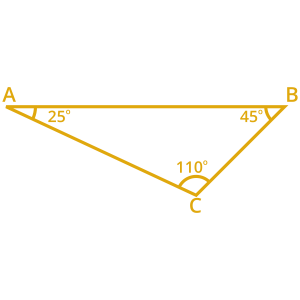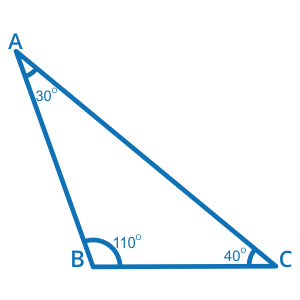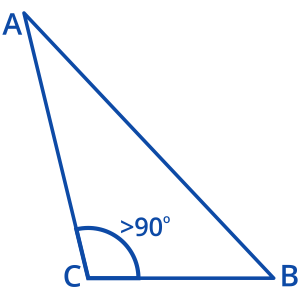### Non-examples of obtuse triangles: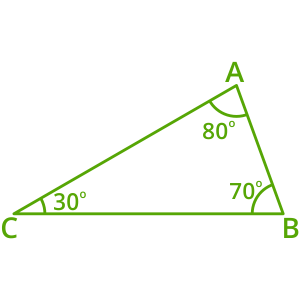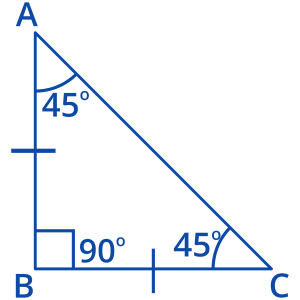### Special facts about obtuse triangle:

• An equilateral triangle can never be obtuse. Since an equilateral triangle has equal sides and angles, each angle measures 60°, which is acute. Therefore, an equilateral angle can never be obtuse-angled.

• A triangle cannot be right-angled and obtuse angled at the same time. Since a right-angled triangle has one right angle, the other two angles are acute. Therefore, an obtuse-angled triangle can never have a right angle; and vice versa.

• The side opposite the obtuse angle in the triangle is the longest.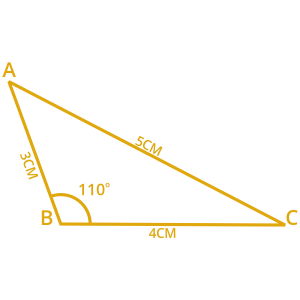### Obtuse angled triangles in real life:

In our surroundings, we can find many examples of obtuse triangles. Here are some examples:

• Triangle shaped roofs

• Hangars found in cupboards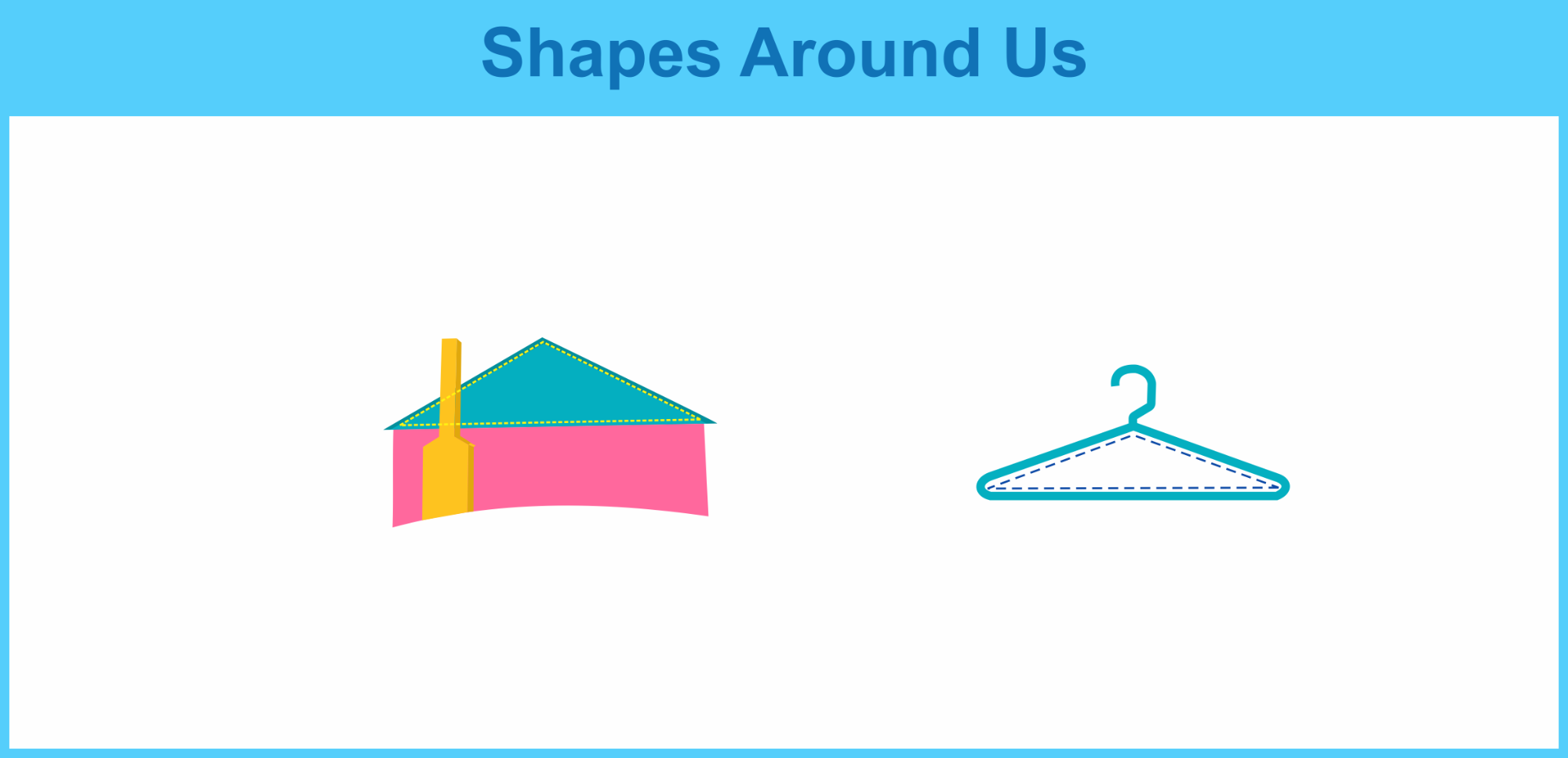Won Numerous Awards & Honors